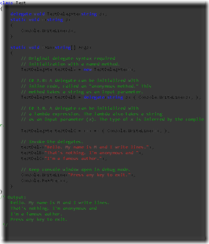Geeks With Blogs

1 What are closures?

1.1 Evolution of Delegates in C#

1.2 Delegates => Closure

2 Captured Variable

2.1 Value of Captured variable

### 1 What are closures?

Closures are function which can be stored in a variable, passed around as parameter and refer to the variables visible at the time they are defined.

#### 1.1 Evolution of Delegates in C#

If we look at the evolution of delegates over the time, it has evolved from standard way of declaration to Anonymous method in C# 2.0, then to lambda expression in C# 3.0.

The following example taken from Anonymous Functions illustrates this#### 1.2 Delegates => Closure

Essentially Delegates satisfy first condition of being closure that it can be treated as First class functions meaning the function logic can be stored as variable, passed as parameter and invoked. Now let us look at the second condition.

Closure should also refer to the variables visible at the time of definition. For this let us look at above example modified

string outerVariable = "Outer Variable";

string capturedVariable = "Captured Variable";

TestDelegate testDelC = (x) => Console.WriteLine(capturedVariable + x);

In the above code, outer Variable “capturedVariable” is referenced by lambda expression.

Please note that “outerVariable” is not referenced by lambda expression so it does not become the Captured Variable (explained below).

So here we see our delegate by referencing outer variable got promoted to Closure.

For further reading please refer to article by Martin Fowler on Closure.

### 2 Captured Variable

Outer variable referenced by the closure is called captured variable. Let us understand the different pitfalls involved with capture variable.

#### 2.1 Value of Captured variable

List<Func<int>> actions = new List<Func<int>>();

int variable = 0;

while (variable < 5)

{

++variable;

}

foreach (var act in actions)

{

Console.WriteLine(act.Invoke());

}

So what is the output of this………..

Many might expect the output to be 0, 2, 4, 6, 8 as the output.

But in contrast it gives 10, 10, 10, 10, 10 as the output. So what went wrong?

The variables captured in the lambda expression in .Net are not constant. Instead they are captured by reference. So when lambda expression is invoked, it takes the value the captured variable has at the time of execution.

To fix this re-write the code like this:

while (variable < 5)

{

int copy = variable;

++variable;

}

Now each time the loop gets executed, we create new instances in copy variable. So now lambda expression refers to individual instances. Now the output of above expression changes to 0, 2, 4, 6, 8 10.

#### 2.2 Lifetime of Captured Variable

Lifetime of captured variable is extended till all the closures referencing the variable become eligible for garbage collection.